## 3.7.3 Expected Travel Distances: The General Case

Our discussion, so far, has focused primarily on exact and approximate expressions for expected travel distances and times to and from incidents in districts with relatively regular ("fairly compact and fairly convex") geometries and uniform distribution of incidents over the districts. Although this focus may appear, at first, to cover only a limited subset of the cases that one may encounter, it turns out that, in practice, our results can be used as "building blocks" to obtain good approximations in a large number of cases where incidents are not uniformly distributed and the district itself does not have a nice rectangular (or circular, triangular, etc.) shape.
Before illustrating this, let us first discuss, in the abstract, the most general possible cases. Let (X, Y) and (X1, Y1,) indicate, respectively, the location of calls for service and of the response unit in a district R of area A. Denote by fx,y,x1,y1 (x,y,x1,y1) the joint pdf for random variables X, Y, X1, and Y1, and by D = d[(X1, Y1,), (X, Y)] the mathematical relationship for the distance between (X1, Y1,) and (X, Y) [e.g., D =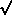[(X1 - X)2 + (Y1 - Y)2] for Euclidean distances]. Then, for the expected travel distance in the district, we have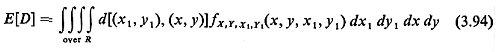Note that the joint pdf for the coordinates of the incident and of the service unit can be made to reflect not only nonuniformities in the distribution over R but also possible dependencies between the locations of incidents and of the service unit.
Expression (3.94) can be extended to the case where N response units are located in district R. Now let (Xi, Yi) indicate the location of the ith response unit (i = 1, 2, . . . , N) and (X, Y) the location of an incident. Then the distance between the incident and the closest response unit can be written

DN = Min {d[(X1, Y1,), (X, Y)], . . . , d[(XN, YN,), (X, Y)]}

Since DN, is then a function of the random variables X, Y, X1, Y1, X2,Y2,. . . . , XN, YN, we can write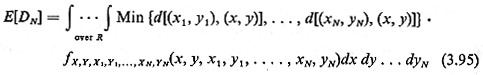where fx, y, x1,y1,...,xn,yn(x,y,x1, ...., yn) is obviously the joint pdf for the coordinates of the incident and the N response units. Thus, in both (3.94) and (3.95) we have expressed expected travel distance as the expected value of a function of random variables whose joint pdf is known. The problem of computing the expected travel distance in the general case is, therefore, no more (or less) difficult than working with any other function of these random variables (cf. Section 3.1). 10
Obviously, in practice, there are severe limitations on how far one can go in deriving such exact expressions for E[D]. Problems become mathematically intractable as the number of random variables increases or as the shape of R and/or the joint pdf for the random variables becomes more complex. In many cases, however, all is not lost as long as one is willing to settle for good approximations rather than exact results. This is true any time the response units are stationary at known locations, no matter what the number, N, of these units is (and for practically any pdf for the spatial distribution of incidents/demands as well as for any shape of the district of interest). It is also true, for any value of N, in the case of mobile response units as long as this approach can also be generalized to expected distances to other than the closest unit (e.g., to the kth closest unit). subdistricts of responsibility have been defined in such a way that each sub-, district of R is served exclusively by a very small number of mobile units (preferably l!). In such instances, the following three-step approach will always work:

STEP 1:  Divide the district.R into several (possibly many) nonoverlapping parts, which we shall call "zones." Each zone must  have the following two properties:

a. Its shape must be approximately rectangular, triangular, circular, or any other easy-to-work-with configuration.

b. The pdf for the spatial distribution of incidents/demands within each zone must be approximately uniform (or that pdf can be approximated by some other sufficiently simple expression as to permit easy mathematical manipulation).

STEP 2: Using the techniques of this chapter, compute all intrazone and zone-to-response unit expected distances, as required  by the problem at hand.

STEP 3: Multiply the expected distances computed in Step 2 by appropriate probabilities to obtain overall expected travel  distances for district R.

Note that each zone in Step I can have an individual shape with its "own" pdf for the distribution of incidents. Note also that the greater the degree of accuracy desired, the larger the number of district zones should be (to approximate better the shape of the district R and the pdf for the spatial distribution of incidents). In fact, the three- step approach outlined above is very similar to the approach that a computer would follow in order to compute numerically the integrals in expressions (3.94) and (3.95).     Rather than attempt a more formal statement of the above three-step approach, we now illustrate it through the following example.

Example 14: Commuter Travel in a Suburban Town

Consider the suburban town shown in Figure 3.31. Its only access to the central business district (CBD) of the metropolitan area of which this town is a part is through the single bridge shown in Figure 3.3 1. The CBD is 6 miles from the bridge's end, as shown. Travel in the town is right-angle, as shown.
We are interested here in the total number of person-miles traveled by the town's working residents (not including schoolchildren) each morning on their way to work. (This. information might be useful in transportation planning or in estimating transportation-related fuel consumption by commuters.)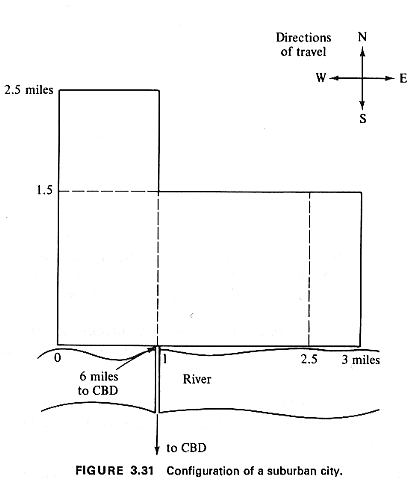About 80 percent of the working residents work in the central city at the CBD. The other 20 percent work in town (and do not have to cross the bridge every morning). Trips are generated uniformly over the town at the rate of about 2,000 trips per square mile. The only exception is the rectangular area to the east of the 2.5-mile mark along the river (as shown in Figure 3.31), where the density of trips generated per square mile decreases linearly according to the function g(d) = 4,000(3 - d), where d is the east-west coordinate (2.5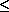or = dor = 3) of each point as measured from the southwesternmost point of the town (see Figure 3.31). There is no difference between the spatial distributions of trip origins to the CBD and to in-town jobs. That is, of every 100 trips generated at each part of the town, no matter where that part is located, 80, on the average, are to the CBD and 20 to in-town jobs.
The spatial distribution (and density per square mile) of in-town jobs is assumed identical to the distribution ( and density) of trip-generating points for in-town jobs. (This may be the case, at least approximately, when there are no concentration of places of employment in a city and when no major employers, such as factories, etc., are located there.) For the purposes of this example, we shall also make the more questionable assumption that the job and residence locations for in-town workers are statistically independent (i.e., that knowledge of where an in-town worker's home is does not affect our a priori knowledge of where in town he or she, and vice versa).

Solution

In working on this problem, we shal first compute the expected travel distance for CBD workers, then the expected travel distance for in-town workers, and finally the total passenger miles covered per day.
To start with, we need a coordinate system. Although our choice of origin does not really make much difference in this case, the edge of the bridge on the town's side is a particularly convenient one. We thus relabel the various points of interest according to this choice of origin, as shown in Figure 3.32a. we can also, using the information given, construct the pdf for the spatial distribution of trip-generation points.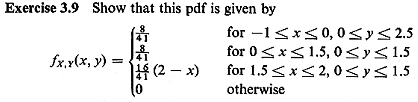Note that fx,y(x,y) also represents the pdf for the spatial distribution of intown jobs according to the problem statement.
With these preliminaries we can now compute:

1. E[D] for CBD workers. Since the coordinates of the edge of the bridge that travelers to the CBD must reach are (0,0), the distance from any point with coordinates (X, Y) to the bridge is given by D = |X| + |Y|.

Exercise 3.10 Show that if we define Z=|X| and W=|Y|, then fz(z) and fw(W) are as shown in Figure 3.32b and c. Note that bothe pdf's can be derived almost by inspection by first obtaining fx(x) and fy(y) from fx,y(x,y). In doing so we use the geometrical probability interpretation of pdf's (cf. Section 3.4.1).
It is now easy to obtain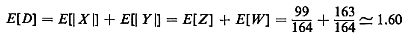miles for the expected distance to point (0,0).

2.  E[D] for in-town workers. we now partition the town into four     non-overlapping zones, as shown in Figure 3.32d. We, wish, in effect, to compute  E[D] between two random points in the town with the locations of each point determined independently, each according to the pdf fx,y(x,y). To do this we consider all possible intrazone and interzone expected distances and then multiply each expected distance by the appropriate probability.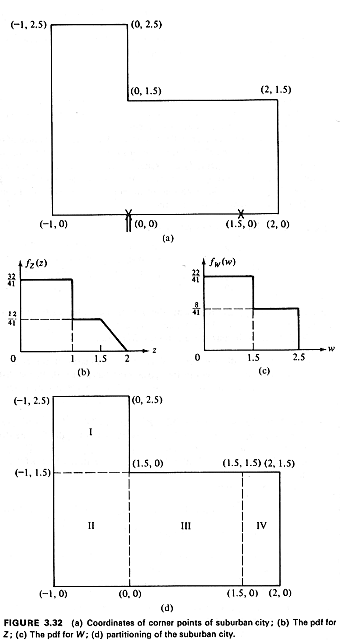For instance, it can be seen that, given an in-town worker:

P{both residence and place of work are in zone I}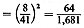E[D | both residence and place of work are in zone I] = 1/3 mile

Exercise 3.11 Show that if both the residence and the place of work of an in-town worker are in zone IV, his or her conditional expected travel distance is equal to 19/30 mile.

Exercise 3.12 By carefully considering all residence and place-of-work combinations, show that for in-town workers, E[D] =~ 1.655 miles.

3. Total expected distance. A total of about 10,250 trips take place every morning. Of those 80 percent (= 8,200) are to the CBD and 20 percent (= 2,050) are in-town. The expected travel distance to a CBD trip is 7.60 miles [remember that point (0,0) is 6 miles from the CBD] while an in-town trip is 1.655 miles long on the average. Therefore, the total expected distance traveled by workers each morning is 65,755 person-miles.

It should be clear that the problem of determining E[D] for CBD workers was equivalent to computing E[D] between an incident distributed as fx,y(x,y) in the city and a fixed service unit located at the CBD. similarly, E[D] for in-town workers is equivalent to the expected travel distance between an incident spatially distributed as fx,y(x,y) in the town and a mobile response unit with that same distribution for its location in the town.
Finally, we might, out of curiosity, wish to compare the result of Exercise 3.12 for the expected travel distance for in-town workers with the result that we could have obtained had we used the approximate expression (3.84) with c=0.67 (Table 3-1), disregarding the fact that the shape of the town of interest is not quite "fairly compact and fairly convex" and that in a part of the town the distributions of demand and the "service unit" (i,e., of the job locations) are not uniform. Since the area of the town is 5.5 square miles, we have E[D]0.675.51.57 miles, for an error of about 5 percent! The reader who worked through Exercises 3.10-3.12 to obtain the exact result of 1.655 will definitely appreciate now the value of approxmate expression (3.84).

10 This approach can also be generalized to expected distances to other than the closest unit (e.g., to the kth closest unit).